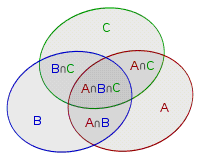# 容斥原理

## 引入## 不定方程非负整数解计数

### 容斥模型

1. 全集 U：不定方程 的非负整数解
2. 元素：变量 .
3. 属性： 的属性即 满足的条件，即 的条件

## HAOI2008 硬币购物

HAOI2008 硬币购物

4 种面值的硬币，第 i 种的面值是 次询问，每次询问给出每种硬币的数量 和一个价格 ，问付款方式。

.

  1 2 3 4 5 6 7 8 9 10 11 12 13 14 15 16 17 18 19 20 21 22 23 24 25 26 27 28 29 30 #include using namespace std; const long long S = 1e5 + 5; long long c, d, n, s; long long f[S]; int main() { scanf("%lld%lld%lld%lld%lld", &c, &c, &c, &c, &n); f = 1; for (long long j = 1; j <= 4; j++) for (long long i = 1; i < S; i++) if (i >= c[j]) f[i] += f[i - c[j]]; // f[i]：价格为i时的硬币组成方法数 for (long long k = 1; k <= n; k++) { scanf("%lld%lld%lld%lld%lld", &d, &d, &d, &d, &s); long long ans = 0; for (long long i = 1; i < 16; i++) { // 容斥，因为物品一共有4种，所以从1到2^4-1=15循环 long long m = s, bit = 0; for (long long j = 1; j <= 4; j++) { if ((i >> (j - 1)) % 2 == 1) { m -= (d[j] + 1) * c[j]; bit++; } } if (m >= 0) ans += (bit % 2 * 2 - 1) * f[m]; } printf("%lld\n", f[s] - ans); } return 0; } 

## 完全图子图染色问题

A 和 B 喜欢对图（不一定连通）进行染色，而他们的规则是，相邻的结点必须染同一种颜色。今天 A 和 B 玩游戏，对于 完全图 。他们定义一个估价函数 ，其中 S 是边集，. 的值是对图 种颜色染色的总方案数。他们的另一个规则是，如果 是奇数，那么 A 的得分增加 ，否则 B 的得分增加 . 问 A 和 B 的得分差值。

## 数论中的容斥

### 容斥原理求最大公约数为 k 的数对个数

 1 2 3 4 for (long long k = N; k >= 1; k--) { f[k] = (N / k) * (N / k); for (long long i = k + k; i <= N; i += k) f[k] -= f[i]; } 

DAG 计数

• 反对称性：若 ，则 ;
• 传递性：若 ，则
• 完全性： 或者

PKUWC2018 随机游走

• 对于 ，有
• 对于 ，有

## 参考文献

Cyhlnj《有标号的 DAG 计数系列问题》

Wikipedia - 全序关系